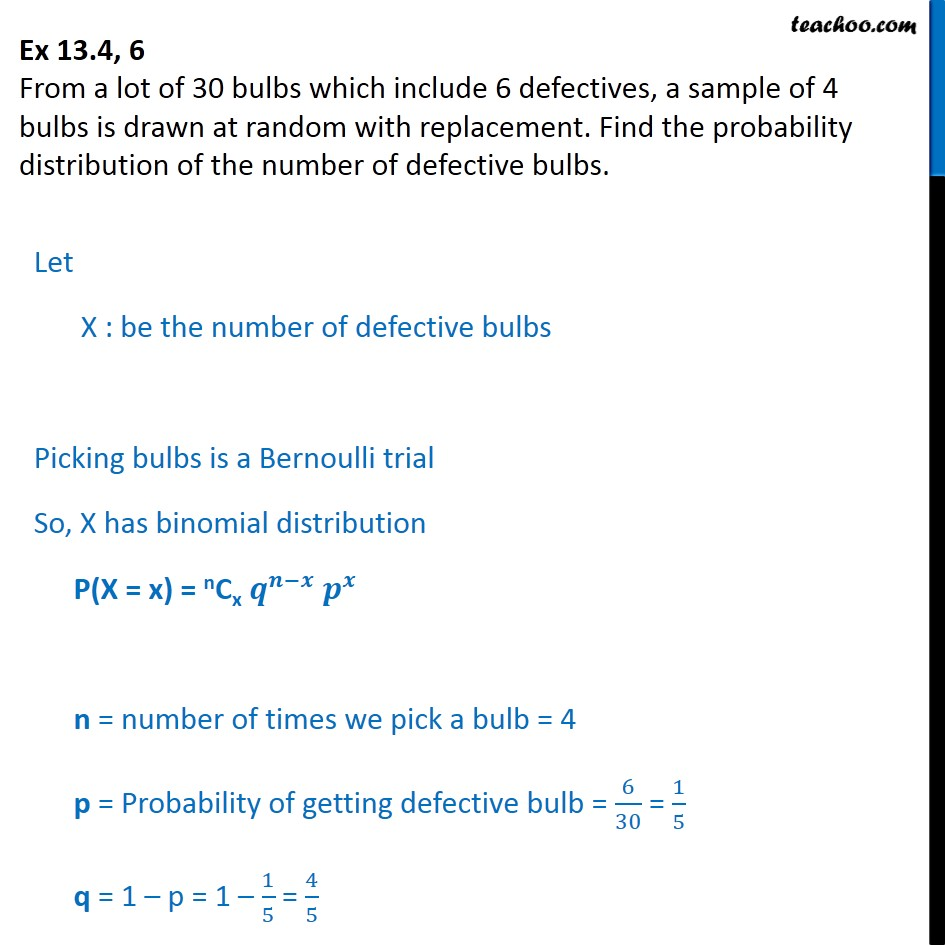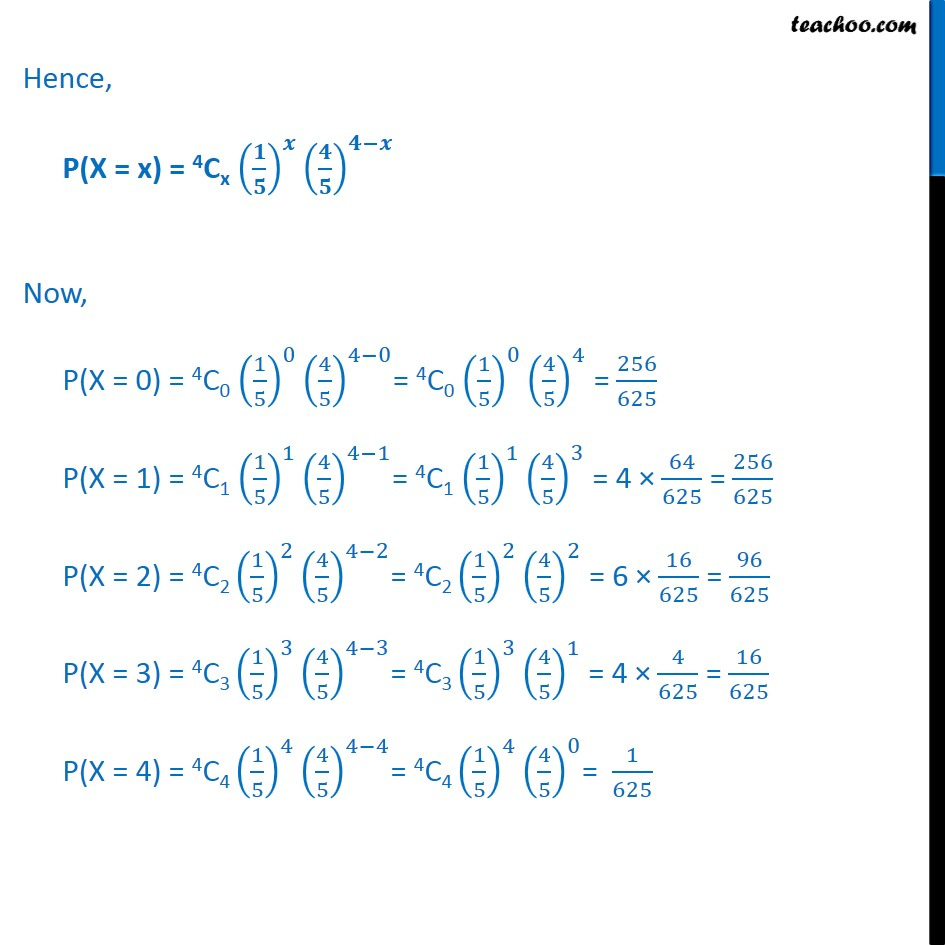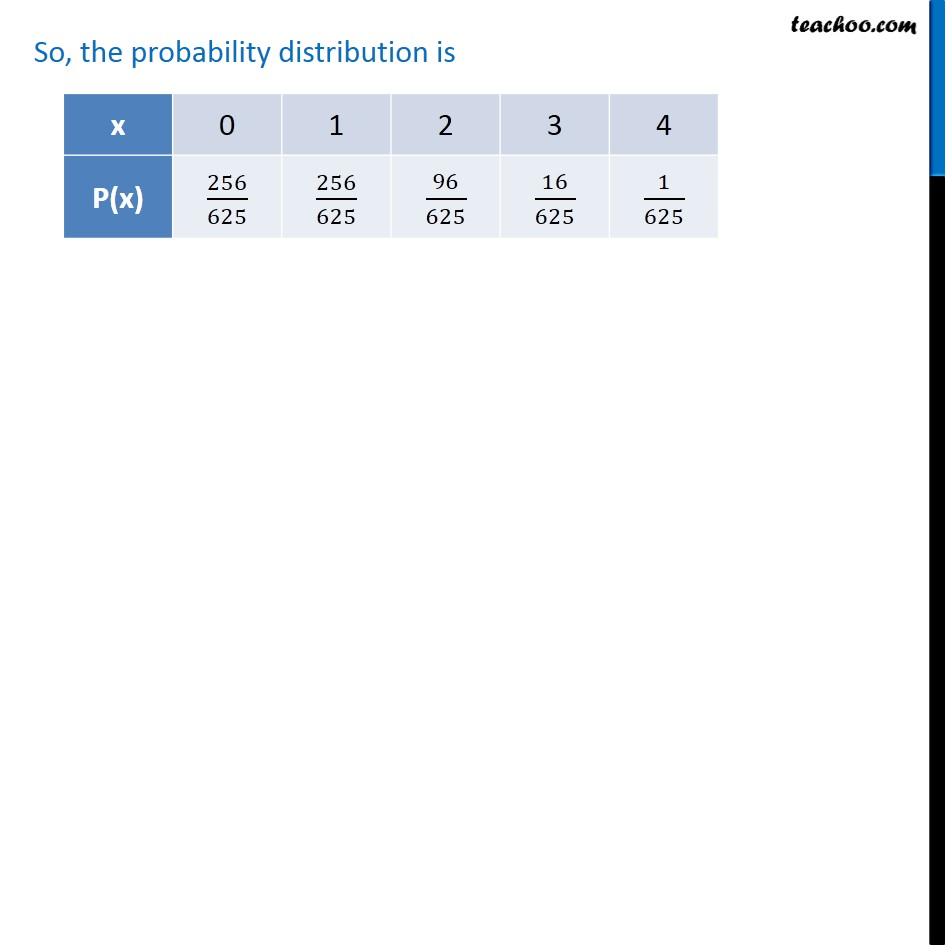1. Class 12
2. Important Question for exams Class 12
3. Chapter 13 Class 12 Probability

Transcript

Ex 13.4, 6 From a lot of 30 bulbs which include 6 defectives, a sample of 4 bulbs is drawn at random with replacement. Find the probability distribution of the number of defective bulbs. Let X : be the number of defective bulbs Picking bulbs is a Bernoulli trial So, X has binomial distribution P(X = x) = nCx 𝒒﷮𝒏−𝒙﷯ 𝒑﷮𝒙﷯ n = number of times we pick a bulb = 4 p = Probability of getting defective bulb = 6﷮30﷯ = 1﷮5﷯ q = 1 – p = 1 – 1﷮5﷯ = 4﷮5﷯ Hence, P(X = x) = 4Cx 𝟏﷮𝟓﷯﷯﷮𝒙﷯ 𝟒﷮𝟓﷯﷯﷮𝟒−𝒙﷯ Now, P(X = 0) = 4C0 1﷮5﷯﷯﷮0﷯ 4﷮5﷯﷯﷮4−0﷯= 4C0 1﷮5﷯﷯﷮0﷯ 4﷮5﷯﷯﷮4﷯ = 256﷮625﷯ P(X = 1) = 4C1 1﷮5﷯﷯﷮1﷯ 4﷮5﷯﷯﷮4−1﷯= 4C1 1﷮5﷯﷯﷮1﷯ 4﷮5﷯﷯﷮3﷯ = 4 × 64﷮625﷯ = 256﷮625﷯ P(X = 2) = 4C2 1﷮5﷯﷯﷮2﷯ 4﷮5﷯﷯﷮4−2﷯= 4C2 1﷮5﷯﷯﷮2﷯ 4﷮5﷯﷯﷮2﷯ = 6 × 16﷮625﷯ = 96﷮625﷯ P(X = 3) = 4C3 1﷮5﷯﷯﷮3﷯ 4﷮5﷯﷯﷮4−3﷯= 4C3 1﷮5﷯﷯﷮3﷯ 4﷮5﷯﷯﷮1﷯ = 4 × 4﷮625﷯ = 16﷮625﷯ P(X = 4) = 4C4 1﷮5﷯﷯﷮4﷯ 4﷮5﷯﷯﷮4−4﷯= 4C4 1﷮5﷯﷯﷮4﷯ 4﷮5﷯﷯﷮0﷯= 1﷮625﷯ So, the probability distribution is

Chapter 13 Class 12 Probability

Class 12
Important Question for exams Class 12